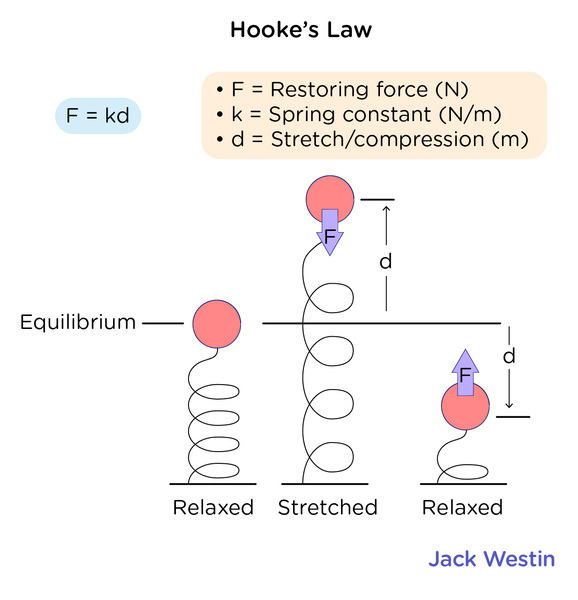Cyber Sale Extended 3 Days: ALL MCAT Products & Admissions Services

### Potential Energy

Topic: Energy Of Point Object Systems

Potential energy (gravitational energy, spring energy, etc)  is the energy difference between the energy of an object in a given position and its energy at a reference position.

Potential energy is often associated with restoring forces such as a spring or the force of gravity. The action of stretching the spring or lifting the mass of an object is performed by an external force that works against the force field of the potential. This work is stored in the force field as potential energy. If the external force is removed the force field acts on the body to perform the work as it moves the body back to its initial position, reducing the stretch of the spring or causing the body to fall. When restoring force does work, the system loses potential energy.

Work of an elastic force is called elastic potential energy; work done by the gravitational force is called gravitational potential energy; and work done by the Coulomb force is called electric potential energy.

Gravitational energy is the potential energy associated with gravitational force, as work is required to move objects against gravity. If an object falls from one point to another point, the force of gravity will do positive work on the object, and the gravitational potential energy will decrease by the same amount. Gravitational potential energy near the Earth can be expressed with respect to the height from the surface of the Earth. (The surface will be the zero point of the potential energy).PE = potential energy measured in joules (J),
m = mass of the object (measured in kg),
h = perpendicular height from the reference point (measured in m),
g = gravitational acceleration (9.8m/s2). Near the surface of the Earth, g can be considered constant.

Spring force is another conservative force, given by the Hooke’s law: F = -kx, where k is spring constant, measured experimentally for a particular spring and x is the displacement. The displacement of spring x is usually measured from the position of “neutral length ” or “relaxed length”. This equation holds true for all forms of oscillatory motion.Practice Questions

Understanding cardiac pressure-volume curves

Basal metabolic rate and energy expenditure

Rollercoasters are energy at work!

MCAT Official Prep (AAMC)

Physics Online Flashcards Question 25

Physics Question Pack Passage 6 Question 36

Physics Question Pack Question 43

Physics Question Pack Question 92

Physics Question Pack Passage 19 Question 111

Practice Exam 2 C/P Section Question 12

Practice Exam 2 C/P Section Passage 10 Question 52

Practice Exam 2 C/P Section Passage 10 Question 53

Practice Exam 4 C/P Section Passage 10 Question 53

Key Points

• Gravitational potential energy near the earth surface: PE = mgh. Near the surface of the Earth, g can be considered constant.

• When a spring is stretched/compressed from its equilibrium position by x, its potential energy is give as U=½ k(x2)

Key Terms

Gravitational acceleration: near the surface of the Earth, g can be considered constant. (9.8m/s^2)

Displacement of spring: measured from the position of “neutral length ” or “relaxed length”

Work: the measure of energy transfer that occurs when an object is moved over a distance by an external force

Potential energy: the energy difference between the energy of an object in a given position and its energy at a reference position

Billing Information